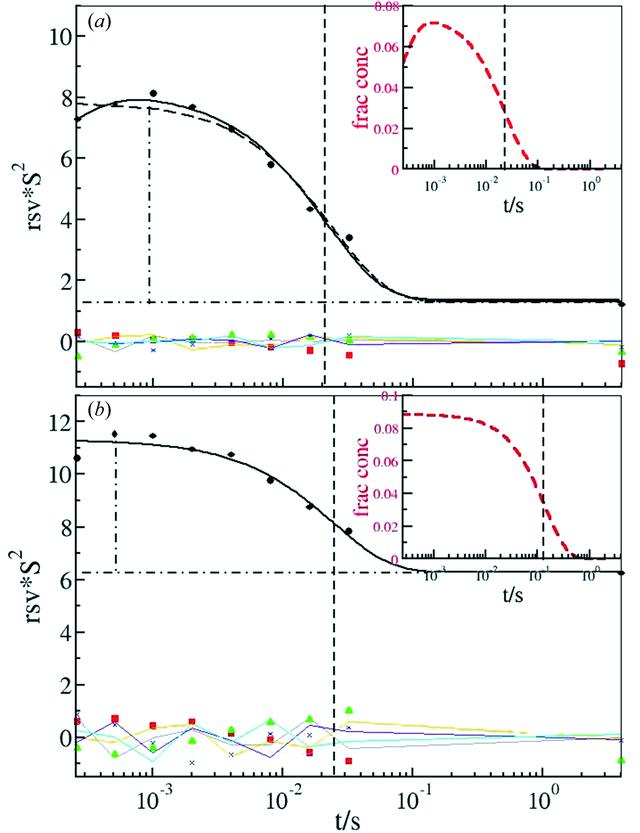disable zoom     view article Figure 5 Right singular vectors (RSV) resulting from the SVD analysis of the short time-series. All singular vectors are shown. (a) First short time-series with the lowest absorbed dose. Solid spheres: first singular vector; solid squares: second singular vector; solid triangles: third singular vector; blue crosses: fourth singular vector. RSV 5 to 8 are shown as thin lines. Solid black line: fit of two exponentials with a source and decaying phase. Vertical dashed line: relaxation time of the decaying phase from a fit of the sum of two exponentials; long dashed line: fit of only one exponential with the same relaxation time; vertical dashed-dotted line: amplitude of RSV1; horizontal dashed-dotted line: offset of RSV1. Insert: red dashed curve: concentration profile of pB1; black dashed line: relaxation time from the inverse of the rate coefficient k2. (b) Tenth short time-series with a high absorbed dose. Solid spheres: first singular vector; solid squares: second singular vector; solid triangles: third singular vector; blue crosses: fourth singular vector. RSV 5 to 8 are shown as thin lines. Vertical dashed line: relaxation time of the decaying phase from fit of only one exponential; vertical dashed-dotted line: amplitude of RSV1; horizontal dashed-dotted line: offset of RSV1. Insert: red dashed curve: concentration profile of pB1; black dashed line: relaxation time from the inverse of the rate coefficient k2.JOURNAL OFSYNCHROTRONRADIATION
ISSN: 1600-5775
Volume 19| Part 2| January 2012| Pages 264-273